#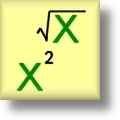Exponents & Radicals Worksheets

## Exponents and Radicals Worksheets for Practice

Here is a graphic preview for all of the Exponents and Radicals Worksheets. You can select different variables to customize these Exponents and Radicals Worksheets for your needs. The Exponents and Radicals Worksheets are randomly created and will never repeat so you have an endless supply of quality Exponents and Radicals Worksheets to use in the classroom or at home. Our Exponents and Radicals Worksheets are free to download, easy to use, and very flexible.

Click the image to be taken to that Exponents & Radicals Worksheet.

#### Exponents PropertiesHandout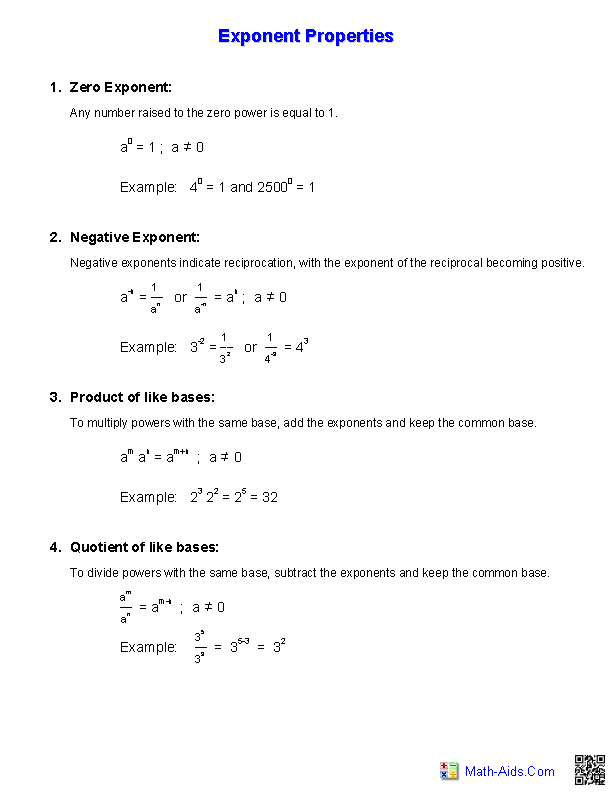#### Square Roots ChartHandout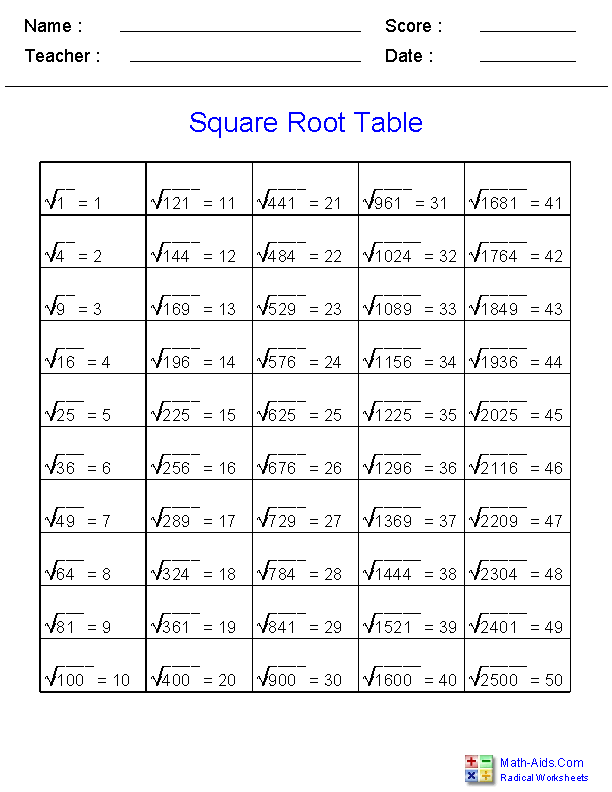#### Simple ExponentWorksheets#### Integers with ExponentWorksheets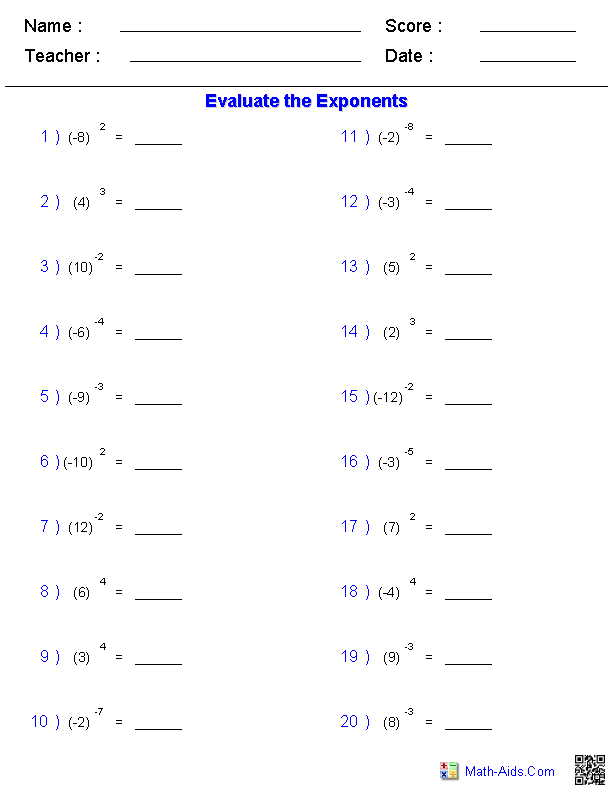#### Fractions with ExponentWorksheets#### Exponents withMultiplication Worksheets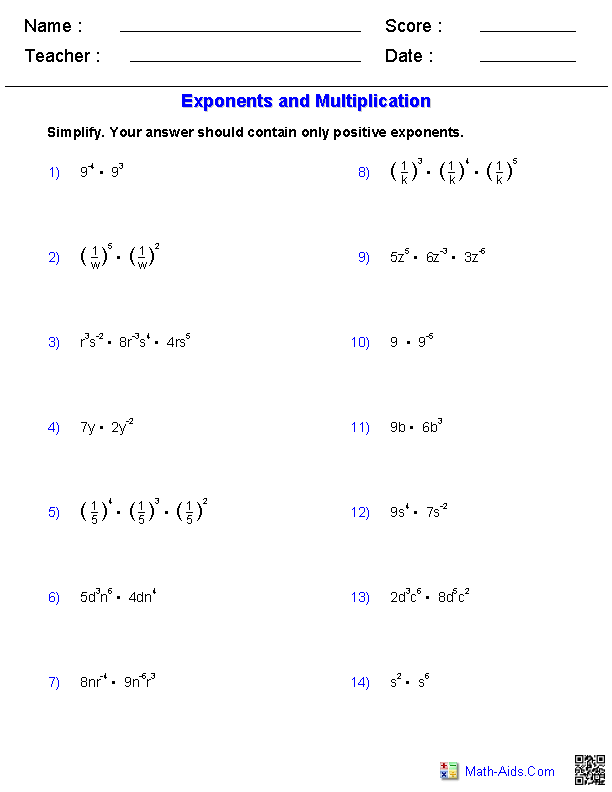#### Exponents withDivision Worksheets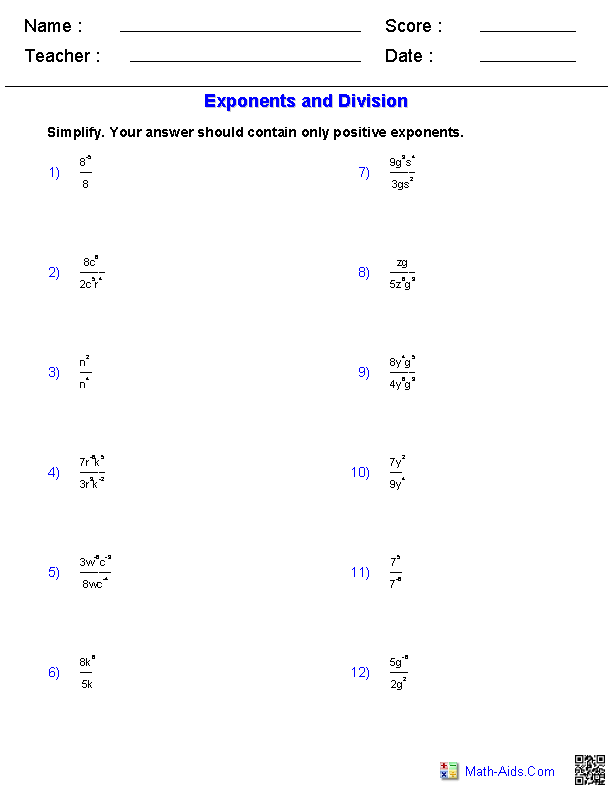#### Exponents withMultiplication & Division#### Powers of ProductsExponents Worksheets#### Powers of QuotientsExponents Worksheets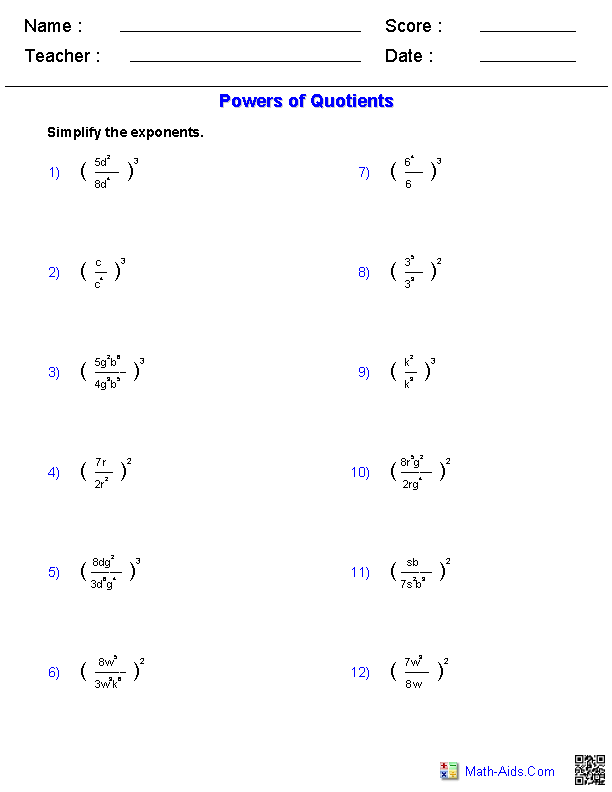#### Powers of Products andQuotients Worksheets#### Evaluating ExponentialFunctions Worksheets#### Operations withExponents Worksheets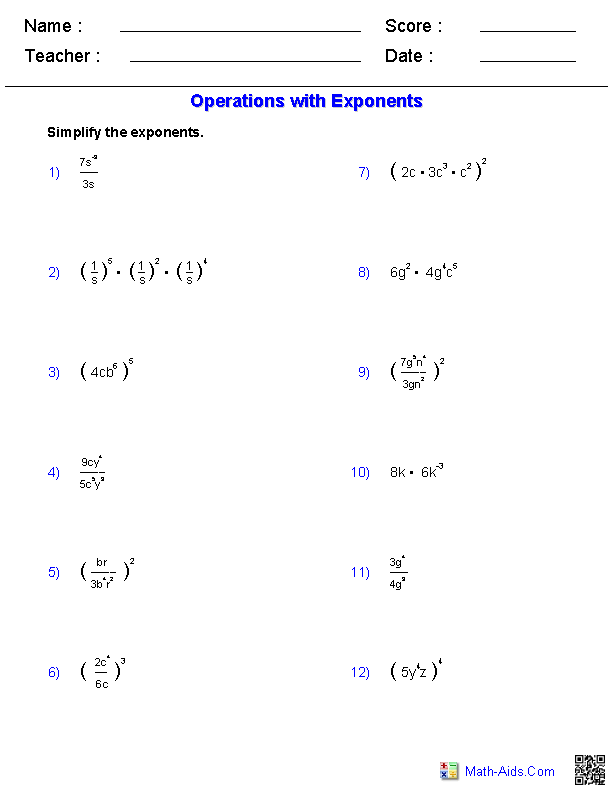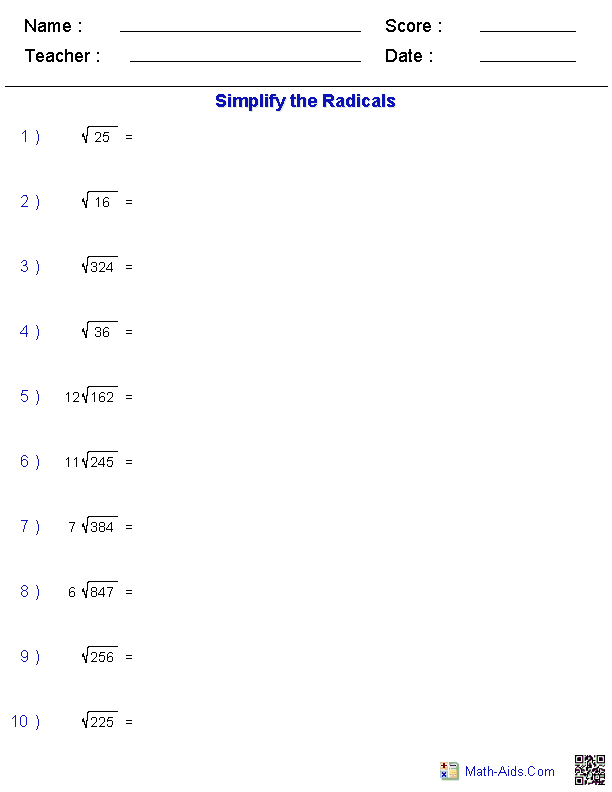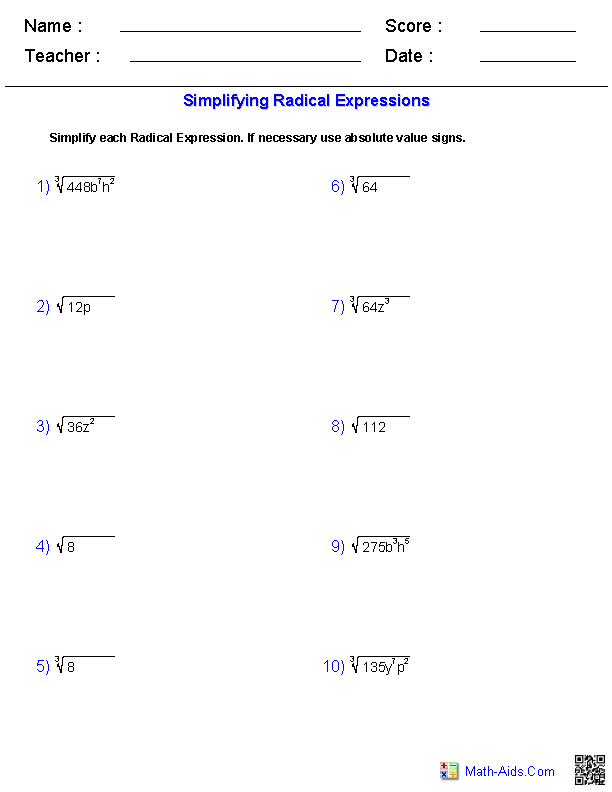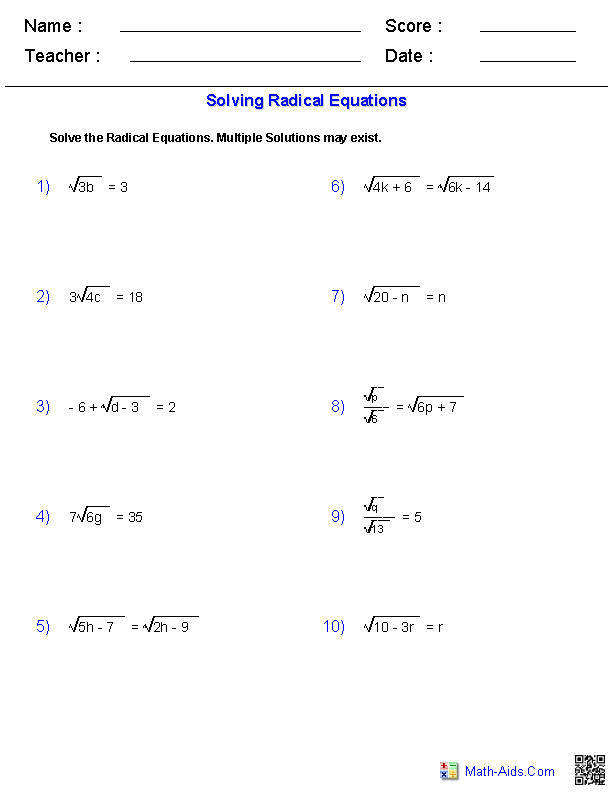#### Scientific NotationWorksheets#### Operations withScientific Notation#### Operations withPerfect Squares and Cubes#### Algebraic Operations withPerfect Squares and CubesRecommended Videos

## Detailed Description for All Exponents & Radicals Worksheets

Exponents Properties Handout
These Exponents Worksheets will produce a handout to define and give examples for the different properties of exponents. These Exponents Worksheets are appropriate for 5th Grade, 6th Grade, 7th Grade, and 8th Grade.

Square Roots Chart Handout
These Radical Worksheets will produce a square roots chart that shows the square roots of the perfect squares from 1 to 50. These Radical Worksheets are appropriate for 5th Grade, 6th Grade, 7th Grade, and 8th Grade.

Integers with Simple Exponent Worksheets
These Exponent Worksheets will produce problems for the introduction of integers with simple exponents. You may select Positive or Negative Bases and ten different Exponents between the range of -4 and +5. These Exponent Worksheets are appropriate for 3rd Grade, 4th Grade, 5th Grade, 6th Grade, and 7th Grade.

Solving Integers with Exponent Worksheets
These Exponent Worksheets will produce problems for practicing how to solve integers with exponents. These Exponent Worksheets are appropriate for 3rd Grade, 4th Grade, 5th Grade, 6th Grade, and 7th Grade.

Solving Fractions with Exponent Worksheets
These Exponent Worksheets will produce problems for practicing how to solve fractions with exponents. These Exponent Worksheets are appropriate for 3rd Grade, 4th Grade, 5th Grade, 6th Grade, and 7th Grade.

Exponents with Multiplication Worksheets
These Exponents Worksheest produces problems for working with Exponents and Multiplication. You may select the problems to contain only positive, negative or a mixture of different exponents. These Exponent Worksheets are appropriate for 5th Grade, 6th Grade, 7th Grade, and 8th Grade.

Exponents with Division Worksheets
These Exponents Worksheets produces problems for working with Exponents and Division. You may select the problems to contain only positive, negative or a mixture of different exponents. These Exponent Worksheets are appropriate for 5th Grade, 6th Grade, 7th Grade, and 8th Grade.

Exponents with Multiplication and Division Worksheets
These Exponents Worksheets produces problems for working with Exponents with Multiplication and Division. You may select the problems to contain only positive, negative or a mixture of different exponents. These Exponent Worksheets are appropriate for 5th Grade, 6th Grade, 7th Grade, and 8th Grade.

Powers of Products Worksheets
These Exponents Worksheets produces problems for working with Products to a Power. You may select the type of problems to use, and this worksheet produces fourteen problems per page. These Exponent Worksheets are appropriate for 5th Grade, 6th Grade, 7th Grade, and 8th Grade.

Powers of Quotients Worksheets
These Exponents Worksheets produces problems for working with Quotients to a Power. You may select the type of problems to use, and this worksheet produces 12 problems per page. These Exponent Worksheets are appropriate for 5th Grade, 6th Grade, 7th Grade, and 8th Grade.

Powers of Products and Quotients Worksheets
These Exponents Worksheets produces problems for working with Products and Quotients to a Power. You may select the type of problems to use, and this worksheet produces 12 problems per page. These Exponent Worksheets are appropriate for 5th Grade, 6th Grade, 7th Grade, and 8th Grade.

Evaluating Exponential Functions Worksheets
These Exponents Worksheets produces problems for evaluating Exponential Functions. You may select the problems to contain only positive, negative or a mixture of different exponents. These Exponent Worksheets are a good resource for students in the 5th Grade, 6th Grade, 7th Grade, and 8th Grade.

Operations with Exponents Worksheets
These Exponents Worksheets produces problems for working with different operations with exponents. You may select from exponents with multiplication or division and products or quotients to a power. These worksheet produces 12 problems per page. These Exponents Worksheets are a good resource for students in the 5th Grade through the 8th Grade.

These Radical Worksheets will produce problems for simplifying radical expressions. You may select what type of radicals you want to use. These Radical Worksheets are a good resource for students in the 5th Grade through the 8th Grade.

These Radical Worksheets will produce problems for adding and subtracting radical expressions. You may select what type of radicals you want to use. These Radical Worksheets are a good resource for students in the 5th Grade through the 8th Grade.

These Radical Worksheets will produce problems for multiplying radical expressions. You may select the difficulty for each expression. These Radical Worksheets are a good resource for students in the 5th Grade through the 8th Grade.

These Radical Worksheets will produce problems for dividing radical expressions. You may select the difficulty for each expression. These Radical Worksheets are a good resource for students in the 5th Grade through the 8th Grade.

These Radical Worksheets will produce problems for solving radical equations. You may select the difficulty for each problem. These Radical Worksheets are a good resource for students in the 5th Grade through the 8th Grade.

Scientific Notation Worksheets
These Scientific Notation Worksheets will produce problems for practicing how to read and write numbers in standard form and scientific notation. These Scientific Notation Worksheets are appropriate for 3rd Grade, 4th Grade, 5th Grade, 6th Grade, and 7th Grade.

Operations with Scientific Notation Worksheets
These Scientific Notation Worksheets produces problems for working with different operations with Scientific Notation. You may select problems with multiplication, division, or products to a power. These worksheets produces 12 problems per page. These Scientific Notation Worksheets are a good resource for students in the 5th Grade through the 8th Grade.

Operations with Perfect Squares and Cubes Worksheets
These Exponents and Radicals Worksheets will produce problems for finding the squares and cubes of positive integers, as well as the square and cube root of perfect squares and perfect cubes. These worksheets produce 18 problems per page. These Exponents and Radicals Worksheets are a good resource for students in the 5th Grade through the 8th Grade.

Algebraic Operations with Perfect Squares and Cubes Worksheets
These Exponents and Radicals Worksheets will produce problems for finding the squares and cubes of algebraic variables, as well as the square and cube root of variables. These worksheets produce 18 problems per page. These Exponents and Radicals Worksheets are a good resource for students in the 5th Grade through the 8th Grade.